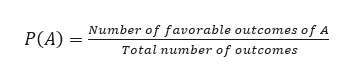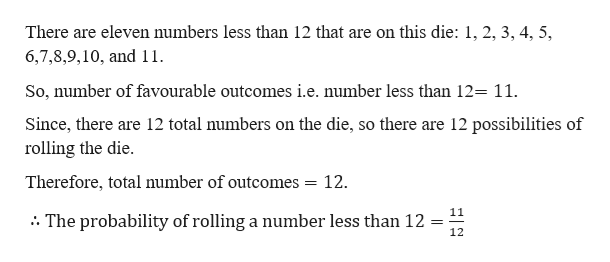A twelve-sided die with sides labeled 1 through 12 will be rolled once. Each number is equally likely to be rolled.What is the probability of rolling a number less than 12?

Question

A twelve-sided die with sides labeled 1 through 12 will be rolled once. Each number is equally likely to be rolled.

What is the probability of rolling a number less than 12?

Step 1

Given,

A twelve-sided die with sides labelled from 1 to 12 will be rolled once. Each number is equally likely to be rolled.

Step 2

We know that,help_outlineImage TranscriptioncloseP(A)Number of favorable outcomes of A Total number of outcomes fullscreen
Step 3

Calculati...help_outlineImage TranscriptioncloseThere are eleven numbers less than 12 that are on this die: 1, 2, 3, 4, 5, 6,7,8,9,10, and 11 So, number of favourable outcomes i.e. number less than 12= 11. Since, there are 12 total numbers on the die, so there are 12 possibilities of rolling the die Therefore, total number of outcomes = 12. 11 The probability of rollinga number less than 12 = 12 fullscreen

Want to see the full answer?

See Solution

Want to see this answer and more?

Our solutions are written by experts, many with advanced degrees, and available 24/7

See Solution
Tagged in

Algebra